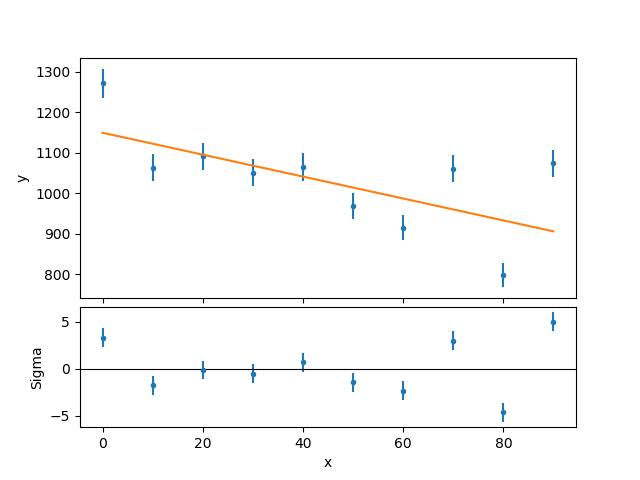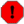# Running Scripts in Sherpa## Overview

#### Synopsis:

This thread lists the available options for running scripts of Sherpa commands. In this thread we refer to a "script" as an ASCII file containing a list of Python Sherpa commands.

Last Update: 5 Dec 2022 - Updated for CIAO 4.15

## Running scripts in Python

You can run scripts (sets of Python commands) directly, or from within Sherpa, as shown below. The main difference is whether or not you want to remain within the Sherpa interactive environment after running the commands.

In the following we will use this script as an example:

```unix% cat example.py
# Use the same seed so the different runs create the same results
np.random.seed(101)

# Create randomized data
x = np.arange(0, 100, 10)
y = np.random.normal(loc=1e3, scale=100, size=x.size)

# Set up model (it isn't the same as used to create the data)
set_source(1, polynom1d.mdl)
thaw(mdl.c1)

# Fit and display results
fit(1)
covar(1)
plot_fit_delchi(1)
plt.savefig('example.png')

# Display the fit results to the screen
cr = get_covar_results()
print("")
print("---- results ----")
for (n, f, l, h) in zip(cr.parnames, cr.parvals, cr.parmins, cr.parmaxes):
print(" {:10s} = {:7.2f}  ({:+8.2f} to {:+8.2f})".format(n, f, l, h))
```

The script creates a randomized data set—using one of the many routines from the NumPy random sampling module—fits it, calculates the parameter errors, and then plots the results, writing them to the file example.png. The final step is to display a formatted version of the best-fit results.

Note that the random number generator is set to use a fixed seed, so that the results below are the same, however the code is run. The np.random.seed line would be removed before using this for data analysis!

Although the suffix .py is commonly used to refer to a Python script, it is not required that scripts use this scheme.

Although not shown in this example, the script can contain, and call, Python function definitions, or import and use other modules.Plot Window not Opening

If a Sherpa plot* function is invoked and fails to display a plot window, include a matplotlib pyplot plt.show() line in your script.

## From the command line

If you just want to run the commands and return to the command lines you can either use the batch mode of Sherpa or use Python to run the commands.

### Sherpa's batch mode

The -b command-line flag for Sherpa turns on batch mode: this means that the starting banner is not displayed and that the interactive mode is not entered. Here is an example of running the above script:

```unix% sherpa -b example.py
Using matplotlib backend: TkAgg
Dataset               = 1
Method                = levmar
Statistic             = chi2gehrels
Initial fit statistic = 9716.47
Final fit statistic   = 77.1754 at function evaluation 15
Data points           = 10
Degrees of freedom    = 8
Probability [Q-value] = 1.80694e-13
Reduced statistic     = 9.64693
Change in statistic   = 9639.29
mdl.c0         1149.13      +/- 20.2657
mdl.c1         -2.70027     +/- 0.369379
Dataset               = 1
Confidence Method     = covariance
Iterative Fit Method  = None
Fitting Method        = levmar
Statistic             = chi2gehrels
covariance 1-sigma (68.2689%) bounds:
Param            Best-Fit  Lower Bound  Upper Bound
-----            --------  -----------  -----------
mdl.c0            1149.13     -20.2657      20.2657
mdl.c1           -2.70027    -0.369379     0.369379

---- results ----
mdl.c0     = 1149.13  (  -20.27 to   +20.27)
mdl.c1     =   -2.70  (   -0.37 to    +0.37)
```

and the PNG version of the plot (example.png, Figure 1) has been created in the working directory.

### Figure 1: Best fit and residuals### Figure 1: Best fit and residualsPython modules

Since we used Sherpa to evaluate the script, we did not have to load in the Sherpa module, and could assume that the NumPy module was loaded as np and that the matplotlib.pyplot module was loaded as plt.

### Python

The script can be run by explicitly calling Python, or by setting it up as an executable script.

However, before we can do this, we need to modify the script to load in the necessary Python modules. The following lines should appear at the start of the script:

```import numpy as np
import matplotlib.pyplot as plt
from sherpa.astro.ui import *
```

At this point you may also need to load in other modules that your script uses, such as pycrates.

With these changes, you can run the script (here called example2.py):

```unix% python example2.py
... same output as above ...
```

If you want to run the script as an executable, then you need to make further changes:

1. the first line of the script should say (before the Python imports):

`#!/usr/bin/env python`
2. the script should be made executable:

`unix% chmod u+x example3`

Note that it is not necessary to drop the .py suffix.

which allows you to do:

```unix% ./example3
... same output as above ...
```

## Within Sherpa

Here we want to remain within the Sherpa interactive environment after executing the script.

### Starting with a script

If the -b flag is not given when running Sherpa, then the script will be executed and then the interactive environment will start up:

```unix% sherpa example.py
-----------------------------------------------------
Welcome to Sherpa: CXC's Modeling and Fitting Package
-----------------------------------------------------
CIAO 4.15 Sherpa version 1 Monday, November 21, 2022

Python 3.9.9 (main, Mar 24 2022, 22:02:45)
IPython 8.0.0 -- An enhanced Interactive Python. Type '?' for help.

IPython profile: sherpa
Using matplotlib backend: TkAgg
Dataset               = 1
Method                = levmar
Statistic             = chi2gehrels
Initial fit statistic = 9716.47
Final fit statistic   = 77.1754 at function evaluation 15
Data points           = 10
Degrees of freedom    = 8
Probability [Q-value] = 1.80694e-13
Reduced statistic     = 9.64693
Change in statistic   = 9639.29
mdl.c0         1149.13      +/- 20.2657
mdl.c1         -2.70027     +/- 0.369379
Dataset               = 1
Confidence Method     = covariance
Iterative Fit Method  = None
Fitting Method        = levmar
Statistic             = chi2gehrels
covariance 1-sigma (68.2689%) bounds:
Param            Best-Fit  Lower Bound  Upper Bound
-----            --------  -----------  -----------
mdl.c0            1149.13     -20.2657      20.2657
mdl.c1           -2.70027    -0.369379     0.369379

---- results ----
mdl.c0     = 1149.13  (  -20.27 to   +20.27)
mdl.c1     =   -2.70  (   -0.37 to    +0.37)

sherpa> quit()
```Note

Top-level variables defined in the script, such as cr in example.py, can be accessed after the script has been run.

### From the interactive session

Scripts can also be run at any time within the Sherpa interactive session by using the %run "magic" command.

```sherpa> %run -i example.py
... same output as above
```The existing Sherpa state

Care should be taken when writing a script for an existing Sherpa session to either make sure that the script either sets everything it needs—such as the optimizer and method—in case it has been changed by the user before loading the script, or that such changes are expected (e.g. to see how changing the optimizer changes the results).

## History

 09 Jun 2009 original version 17 Dec 2009 updated for Sherpa 4.2 29 Jun 2010 updated for CIAO 4.2 Sherpa v2: the Sherpa S-Lang interface has been removed. 15 Dec 2010 Sherpa start-up banner updated for CIAO 4.3 15 Dec 2011 Sherpa start-up banner updated for CIAO 4.4 13 Dec 2012 Sherpa start-up banner updated for CIAO 4.5 26 Aug 2013 Rewritten to include an example script. 03 Dec 2013 Sherpa start-up banner updated for CIAO 4.6, outputs to match 4.6 results. 02 Dec 2014 Updated for CIAO 4.7 14 Dec 2015 Updated for CIAO 4.8 05 Dec 2016 Updated for CIAO 4.9 11 Apr 2018 Updated for CIAO 4.10; added %run iPython magic command 11 Dec 2018 Updated for CIAO 4.11 13 Dec 2019 Updated for CIAO 4.12 (replace ChIPS with Matplotlib). 09 Mar 2022 Added note on using plt.show() if plot display fails to open. 05 Dec 2022 Updated for CIAO 4.15